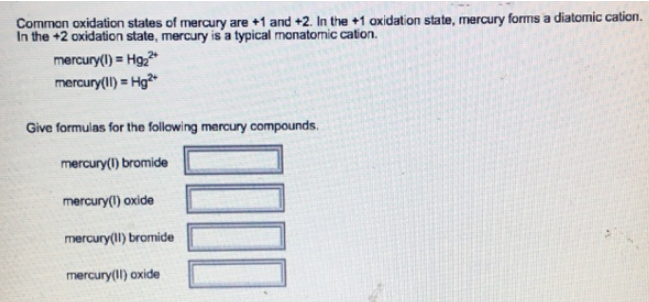# Problem: Common oxidation states of mercury are +1 and +2. In the +1 oxidation state, mercury forms a diatomic cation. In the +2 oxidation state, mercury is a typical monatomic cation. mercury(I) = Hg22+ mercury(II) = Hg2+Give formulas for the following mercury compounds. mercury(I) bromide __________ mercury(I) oxide ____________ mercury(II) bromide _________ mercury(II) oxide ___________

###### FREE Expert Solution
98% (30 ratings)###### Problem Details

Common oxidation states of mercury are +1 and +2. In the +1 oxidation state, mercury forms a diatomic cation. In the +2 oxidation state, mercury is a typical monatomic cation.

mercury(I) = Hg22+

mercury(II) = Hg2+

Give formulas for the following mercury compounds.

mercury(I) bromide __________

mercury(I) oxide ____________

mercury(II) bromide _________

mercury(II) oxide ___________